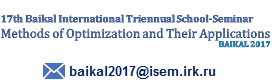## Contact## Section Discrete Optimization

• R. van Bevern
• A parameterized approximation algorithm for the mixed capacitated arc routing problem: Theory and experiments
• V. Bondarenko and A. Nikolaev
• On the skeleton of the pyramidal tours polytope
• P. Borisovsky, A. Eremeev, S. Hrushev, V. Teplyakov and M. Vorozhtsov
• Approximate Solution of Length-Bounded Maximum Multicommodity Flow with Unit Edge-Lengths
• Yu. Chernyshev, A. Sergeyev and A. Ryazanov
• Application of Bioinspired Optimization Artificial Intelligence Technology for Solution Task of Cryptanalysis of Encryption Systems
• I. Davydov and I. Legkih
• A matheuristic for the (r|p)-centroid problem under L1 metric
• A. Eremeev and Yu. Kovalenko
• Genetic Algorithm with Optimal Recombination for Makespan Minimization on Single Machine
• E. Gimadi, I. Rykov and O. Tsidulko
• An Approximation Algorithm for the Euclidean Maximum Connected k-Factor
• M. Golovachev and A. Pyatkin
• On (1, l)-coloring of incidentors of some classes of graphs
• N. Grigoreva
• Approximation Algorithms for Scheduling Problem with Release Times and Delivery Times
• V. Il’ev and S. Il’eva
• On minimizing supermodular set functions on matroids
• A. Kel’manov, S. Khamidullin and V. Khandeev
• A Randomized Algorithm for Two-Cluster Partition of a Sequence
• A. Kel’manov, A. Motkova and V. Shenmaier
• An Approximation Scheme for a Weighted 2-Clustering Problem
• M. Khachay and K. Neznakhina
• Efficient optimal algorithm for the Quasipyramidal GTSP
• K. Kobylkin
• Approximation algorithms for intersecting straight line segments with equal disks
• A. Kononov, M. Kovalyov and Bertrand M.T. Lin
• Minimizing machine assignment costs over Δ-approximate solutions of P||Cmax
• P. Kononova and P. Dolgov
• Local search for multicriteria single machine scheduling with setups
• T. Levanova and A. Gnusarev
• Variable Neighborhood Search Algorithms for Competitive p-Median Facility Location Problem
• Yu. Mezentsev
• Binary Cuts Algorithm for Mixed Integer Programming Problems
• D. Parfenov and I. Bolodueina
• Comprehensive approach to optimization of network resources in a virtual data center
• S. Szabo and B. Zavalnij
• Evaluating heuristics via search tree size estimation
• Yen-Shing Tsai, Bertrand Miao-Tsong Lin and Chih-Chun Lo
• Bin Covering of Subsets
• G. Zabudsky and N. Veremchuk
• Weber Problem on Line with Forbidden Gaps
• L. Zaozerskaya
• On Solving Academic Load Distribution Problem